# AN ENLARGEMENT OF OPERATORS FOR HIERARCHIES

In this section we propose a set of operators which are able to change the primary configuration of a hierarchy by extending or reducing its number of levels, adding a new multiplicity, and creating a multiple hierarchy. To formalize them, let us consider that and are two adjacent levels of a given hierarchy defined as. Let us discuss them.

### Insert level

The Insert level operator makes it possible to add a new level to a hierarchy (giving the variable name, the domain instances, and the relationships between this level and, respectively, the higher and lower adjacent levels in the hierarchy). The insertion of a new level, denoted by li, between the above-mentioned levels, is represented through the symbol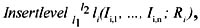, where Ii,1, , Ii,n represents the inserted level domain instances and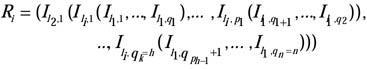(where the instances of levels l2, li, l1, are divided, respectively, into k, h, and n subsets and pj, qv) also represents a generic set of li, and l1 level instances with j = 1,..., h and v = 1, , n.

Example 8: Let us consider the Location dimension shown in Figure 6. Let us suppose we insert the variable County between the variables City and State. We have to define its domain values, as well as the mapping relationship between City and County, and between County and State (see Figure 13). This is obtained by the following formula: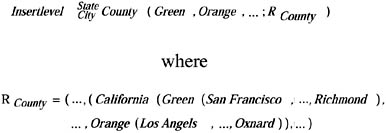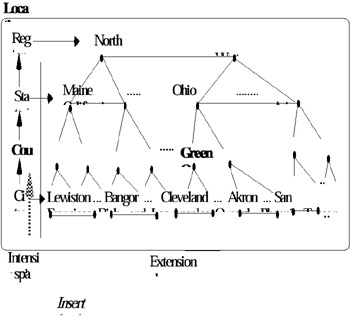Figure 13: Example of an Insert Level

### Delete Level

The Delete level operator redefines a hierarchy as a subset of the existing one by deleting a variable with its relative domain. This operation re-creates the mapping relationships between the two levels (higher and lower) adjacent to the deleted variable. The deletion of a level li is denoted by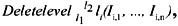, where l1 and l2 are, respectively, the relative lower and higher level in the given hierarchy. This is the converse operation of the insert level operation. Note that in this case the relationships between levels are defined automatically by the system.

The Add multiplicity operator duplicates a given hierarchy, by changing the name of the variables in order to specialize them, as already discussed in a previous section. Let H be a hierarchy. This operator is represented through AddmultiplicityH (additionalname) where additionalname is a string to be added to each variable name of the hierarchy in order to specialize the previous variable name.

Example 9: Let us consider Example 3. The hierarchy City of residence Province of residence Region of residence is obtained by Addmultiplicity Location (of residence)

The Add level operator creates a new hierarchy starting from a given hierarchy. It creates a new variable and its relative domain, and defines the mapping relationship between this and the variable of the starting hierarchy. These two hierarchies define, in this way, a multiple hierarchy. Let ln be a level to be added to a hierarchy defined as l1 12 n1. The Add level operator is represented through: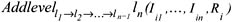and gives, as result, a hierarchy 11 12 1n1 1n

Example 10: An example of a multiple hierarchy has been already shown in Figure 9(d).Multidimensional Databases: Problems and Solutions
ISBN: 1591400538
EAN: 2147483647
Year: 2003
Pages: 150